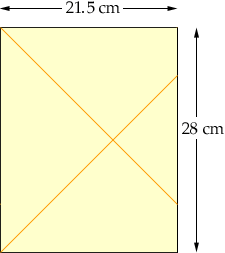Peggy Allan Middle School Grade 6 parent My son has been challenged with "the problem of the week" and I am unable to assist him in finding a reasonable solution. Problem 1, Julio needs to draw a line segment 15cm long. He does not have a ruler. He does have some sheets of letter size paper 28 X 21.5 cm. Describe how Julio can use the paper to measur 15 cm. Note: I have thought of foldinmg the paper in half to obtain 14 cm's but how do you determine 1 cm? Problem 2. This problem is extremely interesting as all the children in the class came to the same conclusion but were but were told it was wrong. See below and advise where the children went wrong. Shopping Spree Greg and Samantha spent a total of seventeen dollars. Greg and Pedro spent twenty dollars Pedro and Samatha spent twenty-one dollars. How much did each person spend. The students answer was: Greg spent (8.50 and 10.00) 18.50 Samantha Spent (8.50 and 10.50) 19.00 Pedro spent (10.00 and 10.50) 20.50 The teacher never gave the students the correct answer. My thoughts are the same as the students, however, as the question really does not give specifics for each pair, maybe the answer should have been the students spent on average approximately 19.33 each. Your assistance is very much appreciated. pegs Hi Pegs, I assume that the sheet is 28 cm from top to bottom and 21.5 cm from side to side as in the diagram. Fold the top side of the sheet to the left side. Now, unfold the sheet. Then, fold the BOTTOM side to the left side. Then unfold again.The orange lines in the diagram are the creases that result. Do you see how that helps? Shopping Spree I think that what is meant is this: The amount spent by Greg plus the amount spent by Samantha is seventeen dollars The amount spent by Greg plus the amount spent by Pedro is twenty dollars The amount spent by Petro plus the amount spent by Samantha is twenty one dollars Now with my interpretation, this answer would not make sense: For instance, Greg alone would have spent more money than him and Samantha put together. Do you see a way of solving the problem if my interpretation is correct? Cheers, Claude Go to Math Central